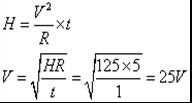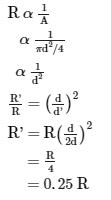Courses

# Test: Heating Effect Of Electric Current

## 15 Questions MCQ Test Science Class 10 | Test: Heating Effect Of Electric Current

Description
This mock test of Test: Heating Effect Of Electric Current for Class 10 helps you for every Class 10 entrance exam. This contains 15 Multiple Choice Questions for Class 10 Test: Heating Effect Of Electric Current (mcq) to study with solutions a complete question bank. The solved questions answers in this Test: Heating Effect Of Electric Current quiz give you a good mix of easy questions and tough questions. Class 10 students definitely take this Test: Heating Effect Of Electric Current exercise for a better result in the exam. You can find other Test: Heating Effect Of Electric Current extra questions, long questions & short questions for Class 10 on EduRev as well by searching above.
QUESTION: 1

### If the current passing through a heater is halved, then the heat produced by it becomes

Solution:

If the current passing through an electric heater has been halved then the heat produced will also be reduced to one fourth because H=I2 RT.

QUESTION: 2

### An electric bulb is marked 18watt-240 volt. If it is used across 240 V power line for one hour daily, calculate the number of days to consume 1 unit of electric energy.

Solution:

Let n be the number of days

Here, work done is 36 x 105 J = (18 J/s) x n x 1 x 60 x 60

Thus, n = 55.5 days

QUESTION: 3

### Two bulbs marked 200 watt-250 volts and 100 watt-250 volts are joined in series to 250 volts supply. Power consumed in circuit is

Solution:

We know that, R = V2 /P

Hence resistance of 1st bulb = (250)2 / 200 = 625/2

resistance of 2nd bulb = (250)2 / 100 = 625

Total resistance of the circuit when the bulbs are connceted in series = 625/2 + 625 = 1875/2 ohm

Therefore, total power consumed in the circuit , P = (250)2 / (1875/2) = 67 watt

QUESTION: 4

Which of the following terms does not represent electrical power in a circuit ?

Solution:

Electric power in terms of current = IR2 and in terms of voltage = V2/R and in terms of voltage and current both = VI

QUESTION: 5

The resistance of a conductor increases with-
I: Increase in length
II: Increase in volume
III: Decrease in area

Solution:
QUESTION: 6

How much heat energy is produced in 10 second in an electric iron having resistance 440 Ω, when a potential difference of 220 volt is applied across it?

Solution:

From ohm’s law, I = V/R = 220/440 = 0.5 A

H = I2 × R × t = (0.5)2 × 440 × 10 = 1100 J

QUESTION: 7

An electric refrigerator rated 500 W operates 10 hr per day. What is the cost of the energy to operate it for 30 days at Rs. 4.00 per kWh ?​

Solution:

E = P × t = 0.5 × 10 × 30 = 150 kWh
Cost of energy = 150 × 4 = Rs. 600

QUESTION: 8

Which property of the electricity is responsible for use of Fuse wire in household wiring?

Solution:

The Fuse wire connection are in between the appliance and main line. It is are very thin wire and when very high current passes through it suddenly, the fuse wire gets heated and melts due to the produced heat. This saves the household appliances

QUESTION: 9

Two light bulbs P and Q are identical in all respects, except that P’s filament is thicker than Q’s. If the same potential difference is applied to each, then

Solution:
Because cross-sectional area is inversely proportional to resistance, so if both bulbs have the same potential difference then, the bulb having more cross-sectional area will have low resistance and glow brighter.

QUESTION: 10

Find the potential difference across the resistor, when 125 J of heat is produced each second in a 5 ohm resistor.

Solution:

H = PtQUESTION: 11

A bulb is rated as 270V, 0.5 A. Its power is

Solution:

Voltage (V) = 270 Volts

Current (I) = 0.5 A

Power (P) = V x I
= 270 x 0.5 = 135 watt

QUESTION: 12

How much energy is transferred when 10 A current flows through a resistor of 5 ohm for 30 minutes ?​

Solution:

P = I2 R = (10)2 × 5 = 500 watt = 0.5 kW

t = 30 minute = 0.5 hr

E = P × t = 0.5 kW × 0.5 hr = 0.25 kWh

QUESTION: 13

Two light bulbs are marked 230 V; 75 W and 230 V; 150 W. If the first bulb has a resistance R, then the resistance of the second is;

Solution:

Power = voltage * current
=  Voltage * ( voltage / resistance)
Hence, if P is power, V is Voltage and R is resistance, then R = V ^2/ R
Hence for first bulb, R1 = V^2/75 = R
For second bulb, R2 = V^2 / 150
= > ½ (V^2/75) = R/2
=> R/2

QUESTION: 14

A certain wire has a resistance R. The resistance of another wire identical with the first and having twice its diameter is;

Solution:

Resistance of a wire is inversely proportional to the cross−sectional area:QUESTION: 15

Commercial unit of electric energy is

Solution:

The commercial unit of electrical energy is kilowatt hour.